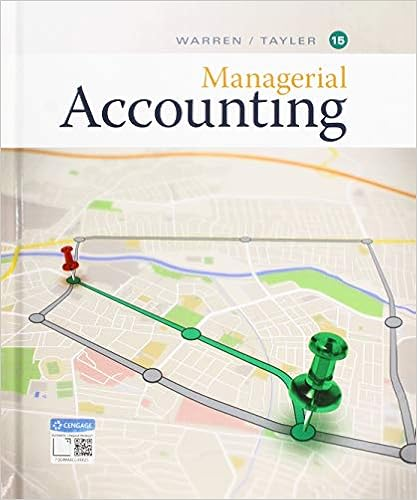# Often as we are solving linear equations we will need

• Homework Help
• 56
• 100% (1) 1 out of 1 people found this document helpful

This preview shows page 11 - 14 out of 56 pages.

##### We have textbook solutions for you!
The document you are viewing contains questions related to this textbook.The document you are viewing contains questions related to this textbook.
Chapter 4 / Exercise 5
Managerial Accounting
Warren/TaylerExpert Verified
Objective: Solve general linear equations with variables on both sides.Often as we are solving linear equations we will need to do some work to set themup into a form we are familiar with solving. This section will focus on manipu-lating an equation we are asked to solve in such a way that we can use our pat-tern for solving two-step equations to ultimately arrive at the solution.One such issue that needs to be addressed is parenthesis. Often the parenthesiscan get in the way of solving an otherwise easy problem. As you might expect wecan get rid of the unwanted parenthesis by using the distributive property. This isshown in the following example. Notice the first step is distributing, then it issolved like any other two-step equation.Example 59.4(2x6)=16Distribute4through parenthesis8x24=16Focus on the subtraction first+24+24Add 24 to both sides8x=40Now focus on the multiply by888Divide both sides by8x=5Our Solution!Often after we distribute there will be some like terms on one side of the equa-tion. Example 2 shows distributing to clear the parenthesis and then combininglike terms next. Notice we only combine like terms on the same side of the equa-tion. Once we have done this, our next example solves just like any other two-stepequation.Example 60.3(2x4)+9=15Distribute the3through the parenthesis6x12+9=15Combine like terms,12+96x3=15Focus on the subtraction first+3 +3Add3to both sides6x=18Now focus on multiply by637
##### We have textbook solutions for you!
The document you are viewing contains questions related to this textbook.The document you are viewing contains questions related to this textbook.
Chapter 4 / Exercise 5
Managerial Accounting
Warren/TaylerExpert Verified
66Divide both sides by6x=3Our SolutionA second type of problem that becomes a two-step equation after a bit of work isone where we see the variable on both sides.This is shown in the followingexample.Example 61.4x6=2x+10Notice here thexis on both the left and right sides of the equation. This canmake it difficult to decide which side to work with. We fix this by moving one ofthe terms withxto the other side, much like we moved a constant term. Itdoesn’t matter which term gets moved,4xor2x,however, it would be theauthor’s suggestion to move the smaller term (to avoid negative coefficients). Forthis reason we begin this problem by clearing the positive2xby subtracting2xfrom both sides.4x6=2x+10Notice the variable on both sides2x2xSubtract2xfrom both sides2x6=10Focus on the subtraction first+6 +6Add6to both sides2x=16Focus on the multiplication by222Divide both sides by2x=8Our Solution!The previous example shows the check on this solution.Here the solution isplugged into thexon both the left and right sides before simplifying.Example 62.4(8)6=2(8)+10Multiply4(8)and2(8)first326=16+10Add and Subtract26=26True!The next example illustrates the same process with negative coefficients. Noticefirst the smaller term with the variable is moved to the other side, this time byadding because the coefficient is negative.
38
Example 63.3x+9=6x27Notice the variable on both sides,3xis smaller+3x+3xAdd3xto both sides9=9x27Focus on the subtraction by 27+27+27Add 27 to both sides36=9xFocus on the mutiplication by999
•••